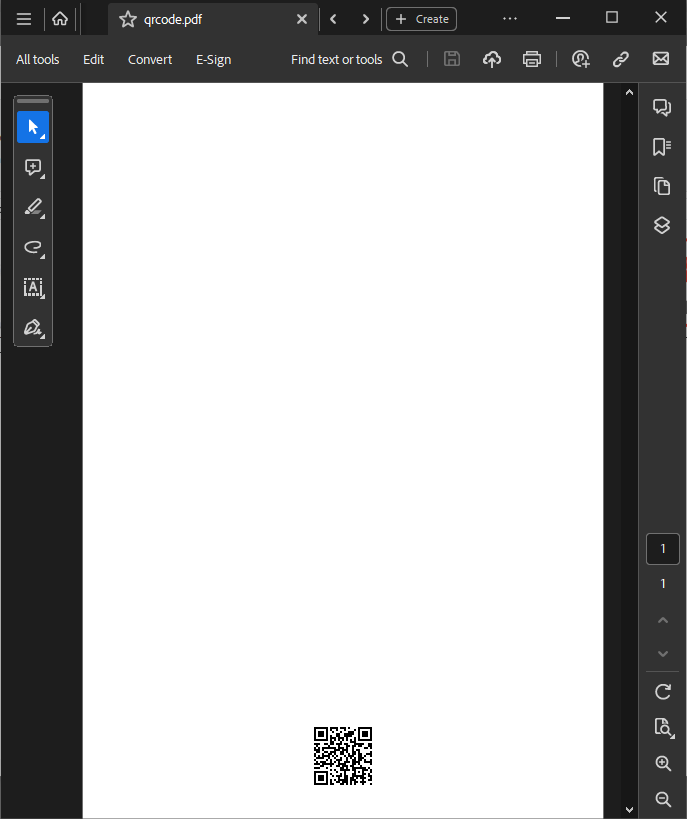# Conversion with scConverter

With scConverter you can add annotations like stamps, text, barcodes, images and much more to the converted output files.
In this tutorial we will look at a few samples on how you can add such annotations, also called markup, to your files.
scConverter provides 3 functions that can add annoations during conversion:

• ConvertMarkup
• scConvertMarkup

Each of these functions accepts a string parameter for markup input.
his string may either be a fully qualified file name, or it may contain markup XML data.
The file may contain binary markup data, which can be created by ViewCompanion and scViewerX, or markup XML data.

Here is how you call the ConvertMarkup function with a markup file name:

ConvertMarkup( "c:\input\in.pdf", "c:\input\stamp.scmx", "c:\output\out.pdf", "PDF", 1.0, 24, 300 );

The stamp.scmx file contains the XML data required to add a stamp to the output file and it looks like the following:

<?xml version="1.0" encoding="UTF-8"?>
<SCMarkupFormat>
<Unit>mm</Unit>
<Elements>
<Element>
<type>Rubber Stamp</type>
<center x="50%" y="50%"/>
<size w="150" h="30"/>
<rotation>45</rotation>
<text>ACCEPTED</text>
<textcolor>#0000FF</textcolor>
<backcolor>#CCCCFF</backcolor>
<font facename='Arial'/>
</Element>
</Elements>
</SCMarkupFormat>

This XML code will add a stamp at center of the page with a rotation of 45 degrees with the text "APPROVED".
As you can see both width and height are set 50% which will place it at the center.
You can optionally put the XML code into a string variable and then call the same function like this:

string MarkupData =
"<?xml version=\"1.0\" encoding=\"UTF-8\"?>\r\n" +
"<SCMarkupFormat>r\n" +
"<Unit>mm</Unit>r\n" +
"<Elements>r\n" +
"<Element>r\n" +
"  <type>Rubber Stamp</type>r\n" +
"  <center x="50%" y="50%"/>r\n" +
"  <size w="150" h="30"/>r\n" +
"  <rotation>45</rotation>r\n" +
"  <text>ACCEPTED</text>r\n" +
"  <textcolor>#0000FF</textcolor>r\n" +
"  <backcolor>#CCCCFF</backcolor>r\n" +
"  <font facename='Arial'/>r\n" +
"</Element>r\n" +
"</Elements>r\n" +
"</SCMarkupFormat>";

ConvertMarkup( "c:\input\in.pdf", MarkupData, "c:\output\out.pdf", "PDF", 1.0, 24, 300 );

Both calls will generate the following output PDF file:### QR Barcode Sample

To add a barcode image to your converted files you may use the following XML (barcode.scmx):

<?xml version="1.0" encoding="UTF-8"?>
<SCMarkupFormat>
<Unit>mm</Unit>
<Elements>
<Element>
<type>Barcode</type>
<barcodetype>QR</barcodetype>
<page>-1</page>
<center x="50%" y="25"/>
<size w="25" h="25"/>
<text>This is a sample text</text>
</Element>
</Elements>
</SCMarkupFormat>

This XML code will add a barcode at center of page width and 25 mm above bottom.
The width and height of the QR image is set to 25mm.
The Page is set to -1, and this will force the annotation element to be added to all pages in a file.
The resulting PDF file will look like the following:The sample XML files used above and more examples, can be found in the SDK Markup XML sample folder:

C:\Users\Public\Documents\ScConverter SDK\Markup XML